Home / Codes / Understanding Pointers | Arduino Programming

# Understanding Pointers | Arduino Programming

Pointers can be confusing to beginner Arduino programmers. This tutorial aims to help you understand the concept of pointers by looking at software and hardware points of view.

For this article, we will limit ourselves to the Arduino UNO board whose main microcontroller is the ATMega328p.The ATMega328p’s memory is as follows:

• Flash Memory 32,000 bytes
• Static Random Access Memory (SRAM) 2000 bytes
• Electrically Erasable Programmable Read-only Memory (EEPROM) 1000 bytes

The flash memory stores the program or sketch. Out of its 32,000 bytes, 500 bytes are for the bootloader. The flash memory and the EEPROM are non-volatile storage areas, meaning, their data remain when the device is off.

The SRAM is volatile memory and is used at the time the sketch is running. This is the variable storage area.

### A Closer Look at SRAM

The ATMega328p’s datasheet shows the data memory like this: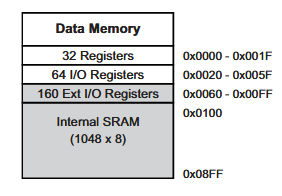For microcontrollers, memory is a stack of registers. The first 32 bytes of the ATMega328p’s data memory are the register file, followed by I/O and extended I/O registers and then the SRAM.
As you can see, the SRAM is from 0x0100 to 0x08FF. That is from 256 to 2,303 in decimals or 2,047 bytes in size.

Now what happens when we declare a variable in Arduino? Depending on the type of variable, a portion of the SRAM is used.

The next question maybe is, which portion of SRAM?

The diagram above is a bit simplified. The image below was produced by the ATMega328p and then sent to a PC via serial (from u/asking_science):### Declaring a Pointer

Simply put, a pointer is a variable that points the address of another variable. A pointer is declared in Arduino like this:

The above statement is useless unless we specify where it points to. Let’s say we want to point the address of an integer variable:

To point the address of i and put that address to p, we do:

Now we are interested as to what is the location of i in SRAM if ever i is the first variable declared. The sketch below prints the address of i in serial monitor:

Run the sketch and this shows in the serial monitor: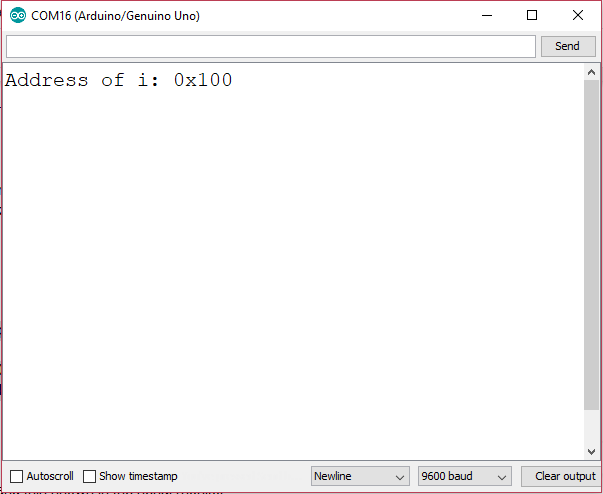This shows that the variable i, an integer type that uses 2-byte space, is in fact stored in the first memory location of SRAM.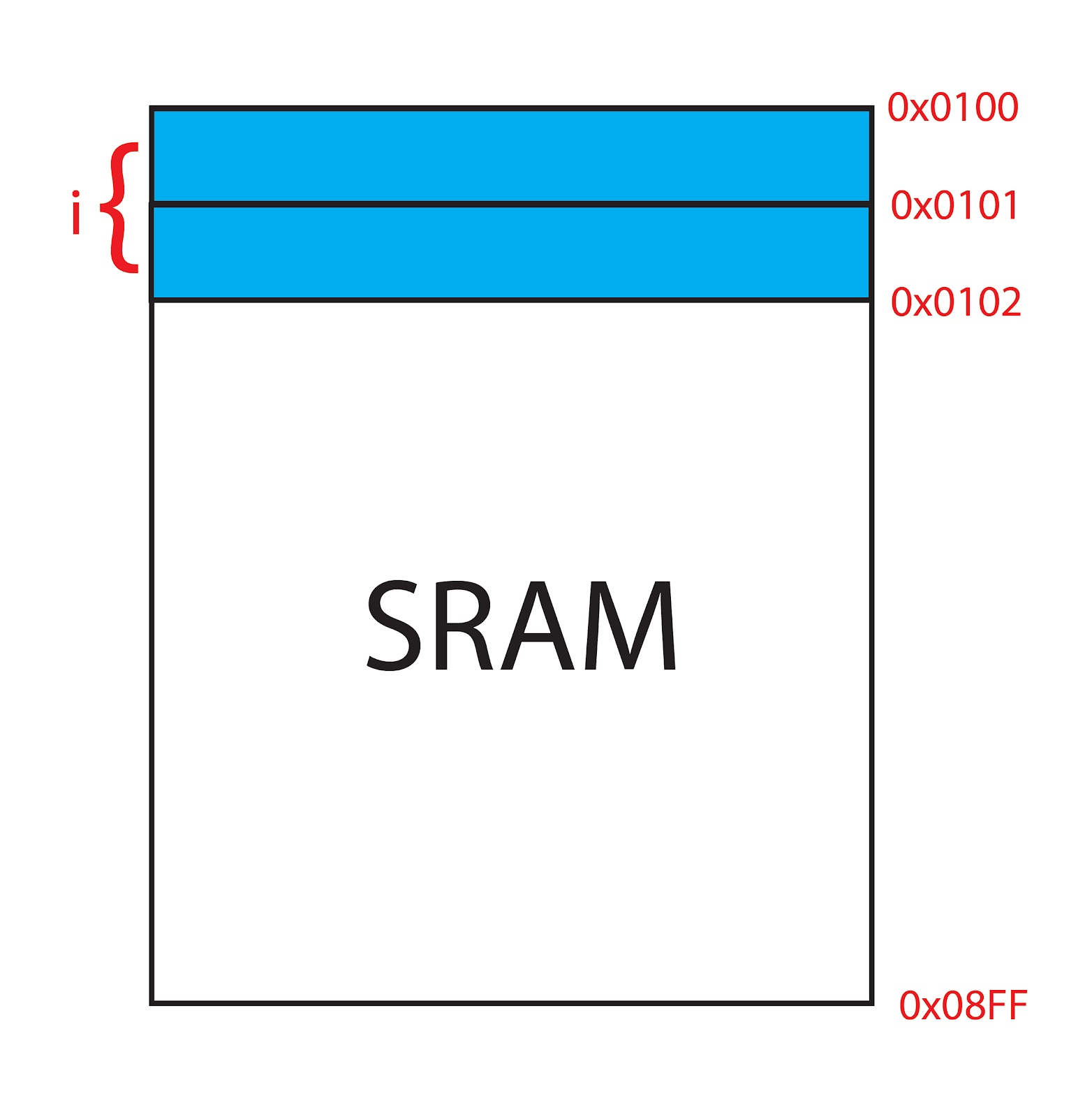Also note that the pointer variable p itself is using a space in the SRAM. To locate the address of p, we can assign another pointer that points at p:

This is casting p to an unsigned 16-bit integer because the Serial.println() function can’t print pointer values directly.

### Using More than One Pointer

If we are to add another variable and another pointer: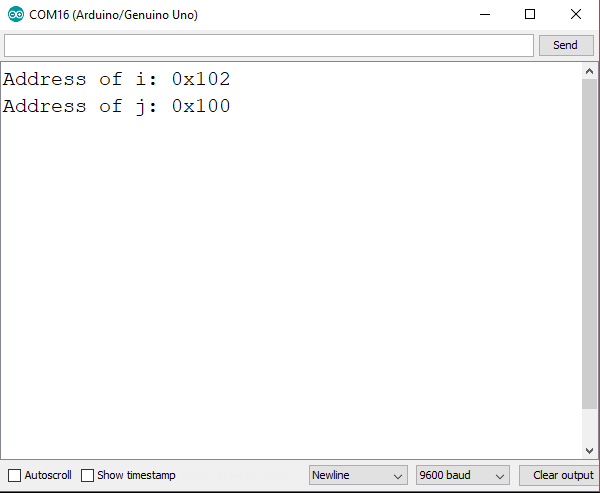Even though that i’s declaration came first before j, j takes the 100H address while i takes the 102H address. This shows the how variables are stored in the SRAM.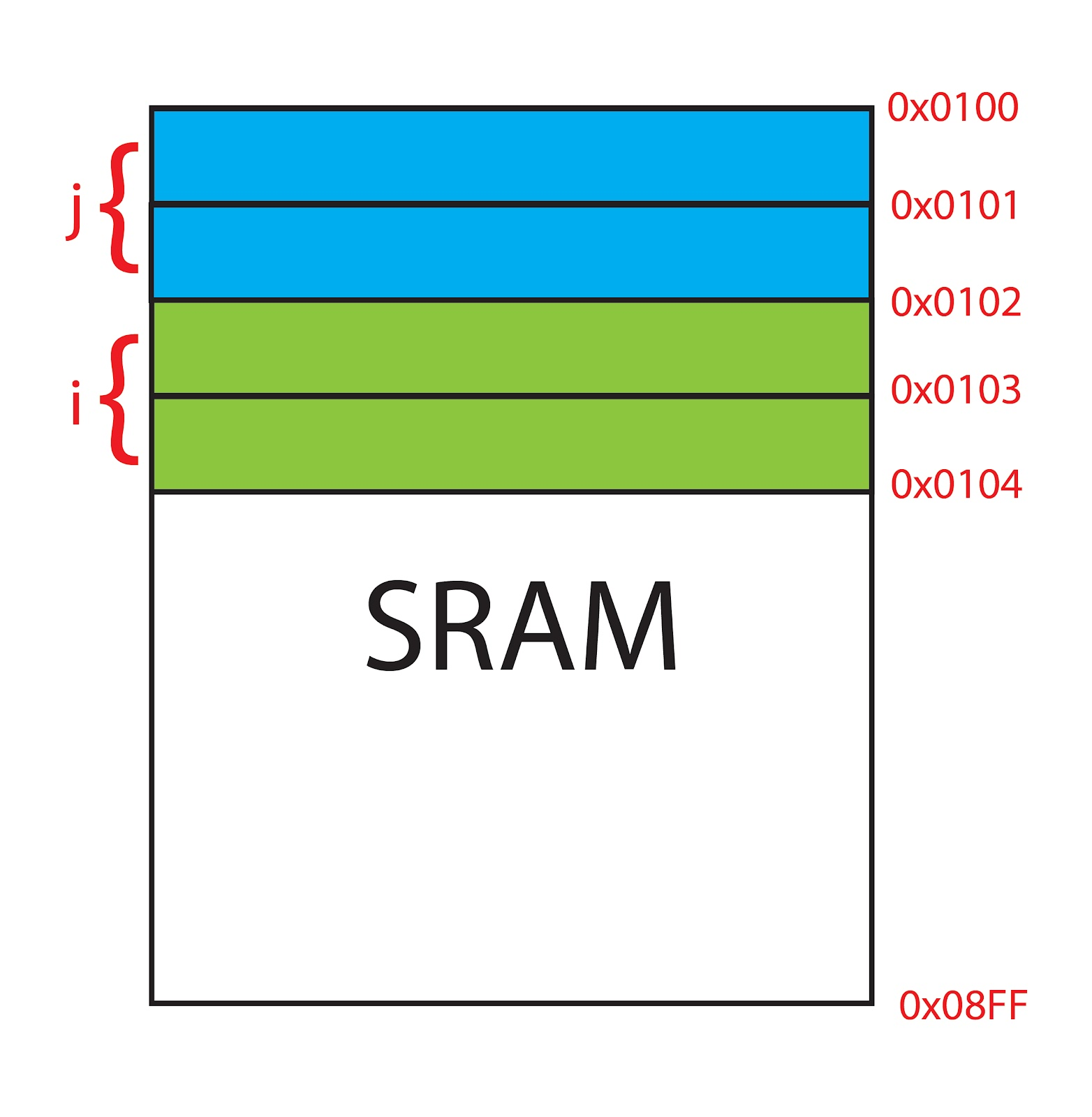Now looking at the Arduino IDE console for the sketch above:

It says that the sketch actually used 196 bytes of SRAM. But we only had four variables right?

• i = 2 bytes
• j = 2 bytes
• *p1 = 2 bytes
• *p2 = 2 bytes

Where is the other 188 bytes?

I tried removing the Serial functions from the sketch and this now shows:

That’s 9 bytes used. This means the Serial functions take 187 bytes!

Where do you think the last extra byte is?

### A Pointer to Array

Let’s say we have an array of integers:

Can we assign a pointer for this array?

If we do it like this:

We will have a compiler error:
This is because i is already the pointer to the array i. To make the above sketch works, we remove the & reference:
The sketch compiles and the serial monitor shows: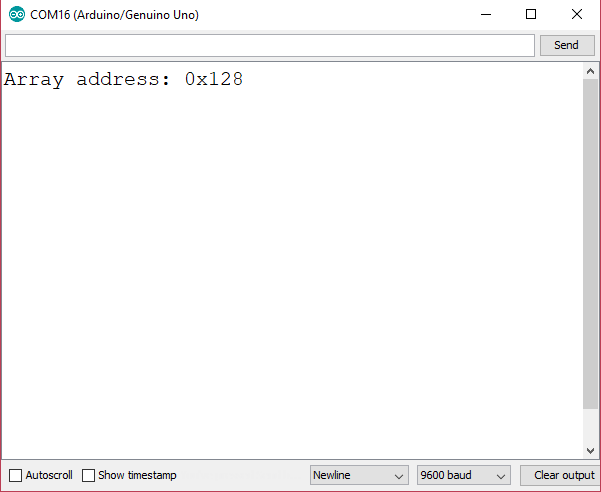Thus, the address of the array i in the sketch above is 128H. It’s interesting as to why the array didn’t occupy the first address of SRAM which is 100H.

Out of curiosity, I tried creating a sketch to show the addresses of all five elements of the array:

The result of this sketch is: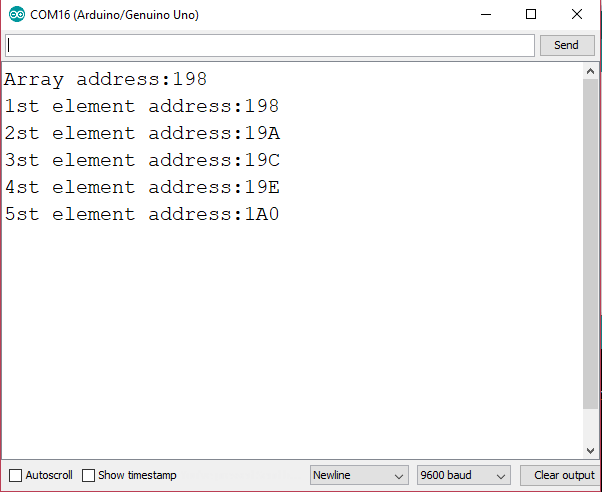Again, the array address changed from 128H to 198H. Also, the 1st element address is equal to the array address.## Calculating Integrals with Arduino

The Arduino platform supports math functions like sine, cosine, logarithm, exponential, etc. But can it …